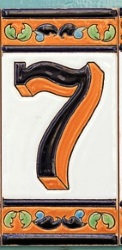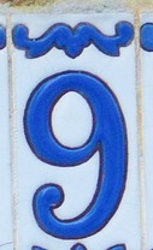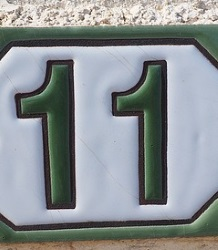#### You may also like### Counting Factors

Is there an efficient way to work out how many factors a large number has?### Summing Consecutive Numbers

15 = 7 + 8 and 10 = 1 + 2 + 3 + 4. Can you say which numbers can be expressed as the sum of two or more consecutive integers?### Helen's Conjecture

Helen made the conjecture that "every multiple of six has more factors than the two numbers either side of it". Is this conjecture true?

##### Age 7 to 14 Challenge Level:
As I walked down the street this morning, I noticed that all of my neighbours' house numbers were odd!I added three house numbers together as I walked past: 7 + 9 + 11 = 27
Further down the road, I passed some bigger numbers. I added another set of three neighbouring house numbers: 15+17+19 = 51

Can you find some other totals I could make, by adding together the house numbers of three (odd) next-door-neighbours?

Once you've found a few totals, here are some questions you might like to explore:

Is there anything special about all the totals?
Is there a quick way to work out the total?
Can you predict what would happen if I walked down the other side of the street instead (where all the houses have even numbers)?

Are there any patterns if I add together four house numbers instead of just three?
Or five house numbers?
Or...

Can you explain and justify the patterns you have noticed?